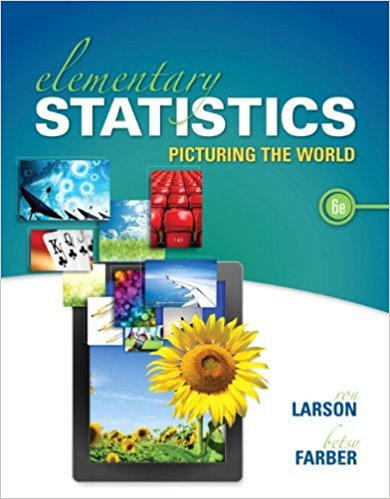×
×

# Solutions for Chapter 7.4: Hypothesis Testing for Proportions## Full solutions for Elementary Statistics: Picturing the World | 6th Edition

ISBN: 9780321911216Solutions for Chapter 7.4: Hypothesis Testing for Proportions

Solutions for Chapter 7.4
4 5 0 319 Reviews
27
2
##### ISBN: 9780321911216

This expansive textbook survival guide covers the following chapters and their solutions. Since 20 problems in chapter 7.4: Hypothesis Testing for Proportions have been answered, more than 118440 students have viewed full step-by-step solutions from this chapter. Chapter 7.4: Hypothesis Testing for Proportions includes 20 full step-by-step solutions. This textbook survival guide was created for the textbook: Elementary Statistics: Picturing the World , edition: 6. Elementary Statistics: Picturing the World was written by and is associated to the ISBN: 9780321911216.

Key Statistics Terms and definitions covered in this textbook
• All possible (subsets) regressions

A method of variable selection in regression that examines all possible subsets of the candidate regressor variables. Eficient computer algorithms have been developed for implementing all possible regressions

• Asymptotic relative eficiency (ARE)

Used to compare hypothesis tests. The ARE of one test relative to another is the limiting ratio of the sample sizes necessary to obtain identical error probabilities for the two procedures.

• Bayes’ theorem

An equation for a conditional probability such as PA B ( | ) in terms of the reverse conditional probability PB A ( | ).

• Box plot (or box and whisker plot)

A graphical display of data in which the box contains the middle 50% of the data (the interquartile range) with the median dividing it, and the whiskers extend to the smallest and largest values (or some deined lower and upper limits).

• C chart

An attribute control chart that plots the total number of defects per unit in a subgroup. Similar to a defects-per-unit or U chart.

• Conidence coeficient

The probability 1?a associated with a conidence interval expressing the probability that the stated interval will contain the true parameter value.

• Conidence level

Another term for the conidence coeficient.

• Control limits

See Control chart.

• Correlation

In the most general usage, a measure of the interdependence among data. The concept may include more than two variables. The term is most commonly used in a narrow sense to express the relationship between quantitative variables or ranks.

• Design matrix

A matrix that provides the tests that are to be conducted in an experiment.

• Discrete random variable

A random variable with a inite (or countably ininite) range.

• Distribution free method(s)

Any method of inference (hypothesis testing or conidence interval construction) that does not depend on the form of the underlying distribution of the observations. Sometimes called nonparametric method(s).

• Distribution function

Another name for a cumulative distribution function.

• Estimate (or point estimate)

The numerical value of a point estimator.

• Exponential random variable

A series of tests in which changes are made to the system under study

• False alarm

A signal from a control chart when no assignable causes are present

• Fisher’s least signiicant difference (LSD) method

A series of pair-wise hypothesis tests of treatment means in an experiment to determine which means differ.

• Fraction defective

In statistical quality control, that portion of a number of units or the output of a process that is defective.

• Generating function

A function that is used to determine properties of the probability distribution of a random variable. See Moment-generating function

• Generator

Effects in a fractional factorial experiment that are used to construct the experimental tests used in the experiment. The generators also deine the aliases.

×